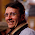## Wednesday, September 22, 2010

### Find the majority

I used this question in my interviews (medium-hard). You have a very long array of integers can you find the int that appears the majority of times with no additional memory and in less than linear time?

1.http://userweb.cs.utexas.edu/~moore/best-ideas/mjrty/index.html should do

2.If an element is majority, it will distributed more than 50% among the total elements. Bifurcating the array for middle value, and conquering for left and right half will yield log(n) elements. A major among these would give us majority.

Generalizing, for example in few constitutions the majority is determined by 2/3 of the total polls. Querying each 2/3 rd element and tracing majority among them would give us the majority :)

Is there any lacuna in my approach?

3.First we need to determine a sample size m << n, then select uniformly at random m ints from the initial array. Then we can use the above algorithm (Bob Boyer) that runs in O(m) time to find the majority element in the sample.

To compute the sample size m we have formulas, so the computation takes constant time.

4.Famous Britney Spears' Problem : http://www.americanscientist.org/issues/pub/the-britney-spears-problem/1

5.there are better solutions.

6.So, the array is sort of available. I assumed it's coming in as a stream and that we're trying to optimize space too.

The solution proposed by Dragos is sublinear and correct with high probability.

Antonio, could you share any of the better solutions?